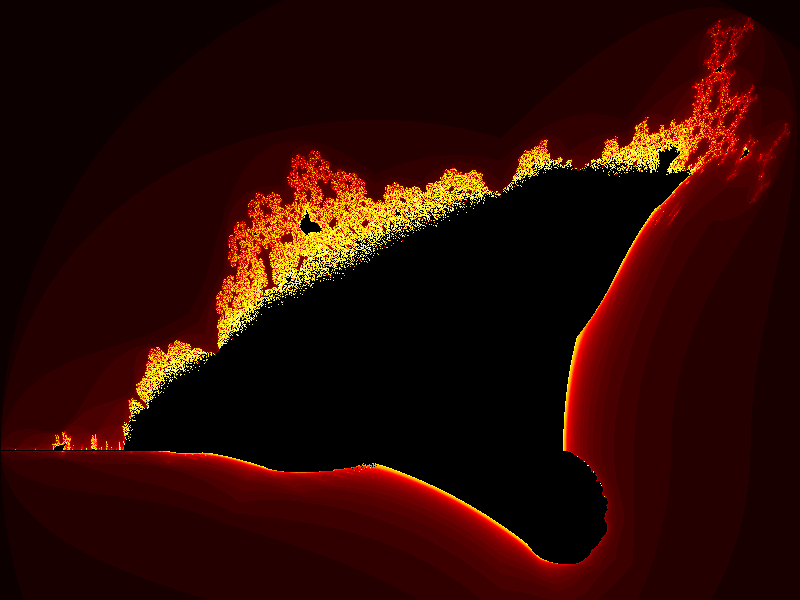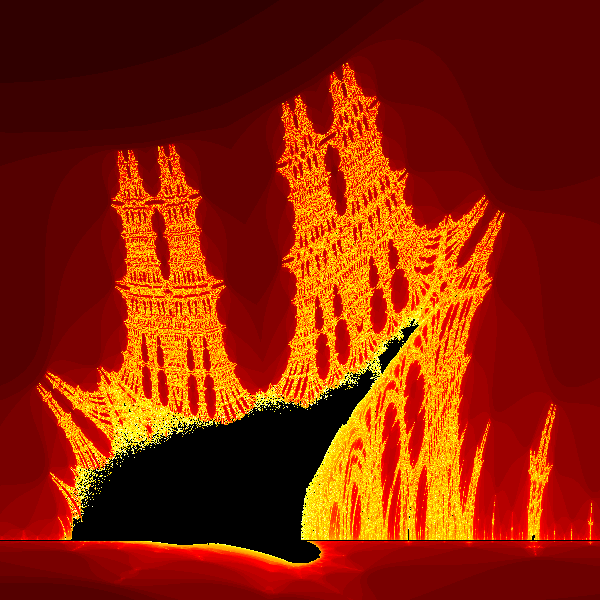# Burning ship fractal with generativepy

Martin McBride, 2021-06-15
Tags burning ship fractal
Categories generativepy generative artThis article has been moved to my blog. Please refer to that article as it might be more up to date.

The burning ship fractal is another famous fractal that can be implemented easily with generativepy.

Like the Mandelbrot set, the burning ship is an escape-time fractal.

## Burning ship formula

The burning ship equations are similar to the Mandelbrot equations:

xnext = x*x - y*y + c1
ynext = 2*abs(x*y) + c2


Where c1 and c2 are two constant values.

The difference compared to the Mandelbrot equations in that the ynext equation uses abs(x*y) rather than just x*y. The absolute value is the positive size of the number, ignoring it's sign. So abs(3) is 3, abs(-3) is also 3.

Here is the image created:## The full code

Here is the full code for the burning ship fractal:

from generativepy.bitmap import Scaler
from generativepy.nparray import (make_nparray_data, make_npcolormap, save_nparray,
from generativepy.color import Color
import numpy as np

MAX_COUNT = 256

def calc(c1, c2):
x = y = 0
for i in range(MAX_COUNT):
x, y = x*x - y*y + c1, abs(2*x*y) + c2
if x*x + y*y > 4:
return i + 1
return 0

def paint(image, pixel_width, pixel_height, frame_no, frame_count):
scaler = Scaler(pixel_width, pixel_height, width=3.2, startx=-2, starty=-1.8)

for px in range(pixel_width):
for py in range(pixel_height):
x, y = scaler.device_to_user(px, py)
count = calc(x, y)
image[py, px] = count

def colorise(counts):
counts = np.reshape(counts, (counts.shape, counts.shape))

colormap = make_npcolormap(MAX_COUNT+1,
[Color('black'), Color('red'), Color('orange'), Color('yellow'), Color('white')],
[16, 8, 32, 128])

outarray = np.zeros((counts.shape, counts.shape, 3), dtype=np.uint8)
apply_npcolormap(outarray, counts, colormap)
return outarray

data = make_nparray_data(paint, 800, 600, channels=1)

save_nparray("/tmp/temp.dat", data)

frame = colorise(data)

save_nparray_image('burning-ship.png', frame)


As well as changing the formula we have also slighlty adjusted the area of the user space (in the call to Scaler), to better fit the image. In adition we have used a red, orange, and yellow colour scheme.

## Zooming in

Just like the Mandebrot set, the burning ship fractal contains miniature "copies" of itself at various places. They aren't actual copies, they have different but similar shapes.

In fact, the small black blob to the left of the main feature is actually more impressive, and is the real reason for the name. Here is what it looks like zoomed in:Toobtain this image, we can use the code above by simple altering the scaler parameters in the paint function:

    scaler = Scaler(pixel_width, pixel_height, width=0.1, startx=-1.8, starty=-0.09)


These parameters zoom in on the new area.

If you found this article useful, you might be interested in the book Computer Graphics in Python or other books by the same author.

#### Popular tags

2d arrays abstract data type alignment and angle animation arc array arrays bar chart bar style behavioural pattern bezier curve built-in function callable object chain circle classes clipping close closure cmyk colour combinations comparison operator comprehension context context manager conversion count creational pattern data science data types decorator design pattern device space dictionary drawing duck typing efficiency ellipse else encryption enumerate fill filter font font style for loop function function composition function plot functools game development generativepy tutorial generator geometry gif global variable gradient greyscale higher order function hsl html image image processing imagesurface immutable object in operator index inner function input installing iter iterable iterator itertools join l system lambda function len lerp line line plot line style linear gradient linspace list list comprehension logical operator lru_cache magic method mandelbrot mandelbrot set map marker style matplotlib monad mutability named parameter numeric python numpy object open operator optimisation optional parameter or pandas partial application path pattern permutations pie chart polygon positional parameter print pure function python standard library radial gradient range recipes rectangle recursion reduce regular polygon repeat rgb rotation roundrect scaling scatter plot scipy sector segment sequence setup shape singleton slice slicing sound spirograph sprite square str stream string stroke structural pattern subpath symmetric encryption template text text metrics tinkerbell fractal transform translation transparency triangle truthy value tuple turtle unpacking user space vectorisation webserver website while loop zip